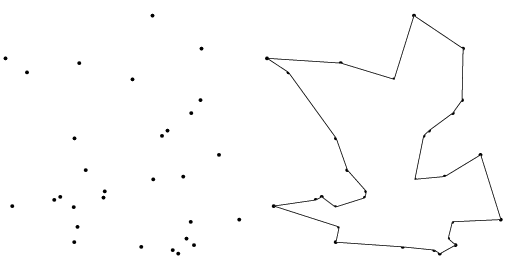## Homework 5 Solving Travelling Salesman Problem Using Pthread

Computer Architecture I ShanghaiTech University
HW4 HW5 HW6

### Travelling Salesman Problem

#### Introduction of TSP

The travelling salesman problem (TSP) asks the following question:

"Given a list of `cities` and the `distances` between each pair of cities,
what is the `shortest` possible route that `visits each city and returns to the origin city`?"(You want to "link" these cities to form a shortest route)

It is actually an NP-complete problem (there's NO polynomial algrithms to solve this problem), It is important in operations research and theoretical computer science and also has many kinds of applications such as planning, logistics, and the manufacture of microchips, etc...

Check this wikipedia link for more details.

#### Problem Setup

Welcome to the NP-complete world, in this homework, you will try to solve this really hard problem use some strategy and accelerate using `pthreads`. You are a travelling salesman, given a map and start point, and you need to find the shortest route which starts at the given point, goes over all these cities only once (except the start city) and finally goes back.

You should implement a travelling salesman problem(TSP) solver in C.

#### Input

The first line of input contains 3 integers `t`,`n` and `s`
• `t` means the number of threads (main thread is not included), ` 0< t <=20 `
• `n` stands for the total number of cities
• `s` stands for the start point, ` 0 <= s < n `
• `n` will be guaranteed to`<= 10` in Autolab testcases.
The next `n` lines represent a symmetric adjacency matrix `A` (because the edges are `undirected`).
`A[i][j]` represents the ` distance `between the cities `i` and `j`, for all valid `i, j`, `1000>=A[i][j]>=0` and they are all `integers`.
A distance of `0` means their is no road between city `i,j`

For example:

``` 2 4 0 0 100 100 100 100 0 35 25 100 35 0 30 100 25 30 0 ```

#### Output

One single integer tells the total length of the route if it's feasible, -1 otherwise. The following example won't necessary be the right answer.
``` 200\n ```

#### Requirement

• You only allowed to inclue: `stdio.h stdlib.h string.h pthread.h`
• We will check memory leak using valgrind.
• Comment Rule. You need to have MEANINGFUL comments no less than 25% of the total lines of code you wrote.
• You must create `t` number of threads and all of them should do `reasonable` things (Otherwilse we will deduct 50% of your points).
• Of course your program should output the `precise` value, so `Randomized Algorithms` and `k-Approximation Algorithms` will be very likely to fail the test cases.
• Your code must stay in 2 files `tsp.c` and `tsp.h`, which will be created by yourself. We will not offer a code template this time.
• We will use the following Makefile:
``````CC=gcc
CFLAGS=-Wpedantic -Wall -Wextra -Werror -std=c89 -pthread

tsp.o: tsp.c tsp.h
\${CC} \${CFLAGS} tsp.c -o tsp.o``````
• Memory limit will be 40960KB in Autolab.
• You can test your memory by type `ulimit -v 40960` in Terminal and then run `./tsp.o < input_file_name `
• .

• Submit to Autolab with a file named hw5.tar, containing exactly two files: `tsp.c tsp.h`, it should produce a tsp.o file using our Makefile and can be tested using this command `./tsp.o < input_file_name `
• Autolab will just test your code with some `small <= 10 ` testcases, to verify the correctness of your program and it will contribute `60%` of the whole points.
• After the autograding deadline, we will run your code on a larger server with higher performance with a bigger testcase to test your speed.
• AC all the testcases in Autolab `DOES NOT` guarentee your correctness in the larger testcases on the bigger server, if you have something wrong with our code and use some randomized Algorithm or approximation algrithms.
• For the other `40%` of points: if you fail on the bigger testcases (like find a sub-optimal sulution), you will got `0` points, for programs which output the right answer, the fastest 20 people will get full points, the top 21~50 will get 80%, top 51~80 get 60%, others get 40%

#### Hints

• Implement a Brute Force Algorithm (Generate and test all the `(n-1)!` permutations of routes) and optimize it using pthread might be a safe and reasonable choice.
• Consider a cleverer inplementation of Brute Force algorithm when you generate permutations. (Generate it online or use recursive search.)
• You can also try to model it as Linear Programming
• Dynamic Programming is fast but you may consider how to deal with data concurrency problem, it's very hard to parallelize.
• Approximation and Randomized Algorithm is not recommanded, but not restricted. Though it is more feasible and much faster in practice but it may find suboptimal sulutions.
• If you implement some advanced algorithms and parallelize it additionaly, you can come to jiangchy & nianzhl's office hour, we may give you some bonus if you really did a good job.